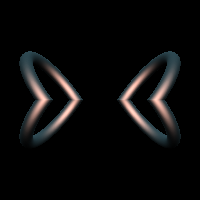filter
RayTK
v0.36

Mirror coordinates across two axes.

This is similar to `mirrorOctant` but without mirroring on the diagonals.

## Parameters

• `Enable`:
• `Axis`: Axis that faces the plane where coordinates are mirrored.
• `YZ`:
• `ZX`:
• `XY`:
• `Size`: Spacing of the reflection planes.
• `Offset`: Shifts the input before applying reflection.
• `Rotate Axis`: Rotates the input before applying reflection.
• `Iteration Type`: Exposes information to upstream operators about which quadrant a point is in.
• `None`:
• `Quadrant Index (0-3)`: Numbers the quadrants from 0 to 3.
• `Signed Axes (-1/1, -1/1)`: Populates the x and y parts of the iteration value with 1 or -1 for different sides of the dividing planes.

## Inputs

• `definition_in`: (optional)
• Coordinate Types: `vec2` `vec3`
• Context Types: `Context` `MaterialContext` `CameraContext` `LightContext` `RayContext` `ParticleContext`
• Return Types: `float` `vec4` `Sdf` `Ray` `Light` `Particle`
• `Rotate Axis Field`: (optional) Value field used to vary the `Rotateaxis`. If the field is a 1D field, it is given the distance from the center. If it is a 2D field, it is given the position along the mirror axes. If it is a 3D field, it is given the raw position. The value is converted to radians and *added* to the `Rotateaxis` parameter.* `offset_field_definition_in`:
• Coordinate Types: `float` `vec2` `vec3`
• Context Types: `Context` `MaterialContext` `CameraContext` `LightContext` `RayContext` `ParticleContext`
• Return Types: `float` `Sdf`
• `Offset Field`: (optional)
• Coordinate Types: `float` `vec3`
• Context Types: `Context` `MaterialContext` `CameraContext` `LightContext` `RayContext` `ParticleContext`
• Return Types: `float` `vec4`

## Variables

• `index`:
• `sign`: